# Violin plot by group in R

Tom Kelly

## Sample data

Consider the `chickwts` data set, which contains a numerical variable giving the chicken weights from an experiment and a categorial variable giving the feed type of each chicken.

``````# Sample data set
df <- chickwts``````

## Violin plot by group

Default violin plots

Violin plots are an alternative to box plots which can be created in base R with `vioplot` package. In order to create a violin plot by group you will need to input a formula, such as `y ~ x`, being `y` a numerical variable and `x` a categorical variable containing groups.

``````# install.packages("vioplot")
library("vioplot")

vioplot(weight ~ feed, data = df)

# Equivalent to:
vioplot(df\$weight ~ df\$feed)``````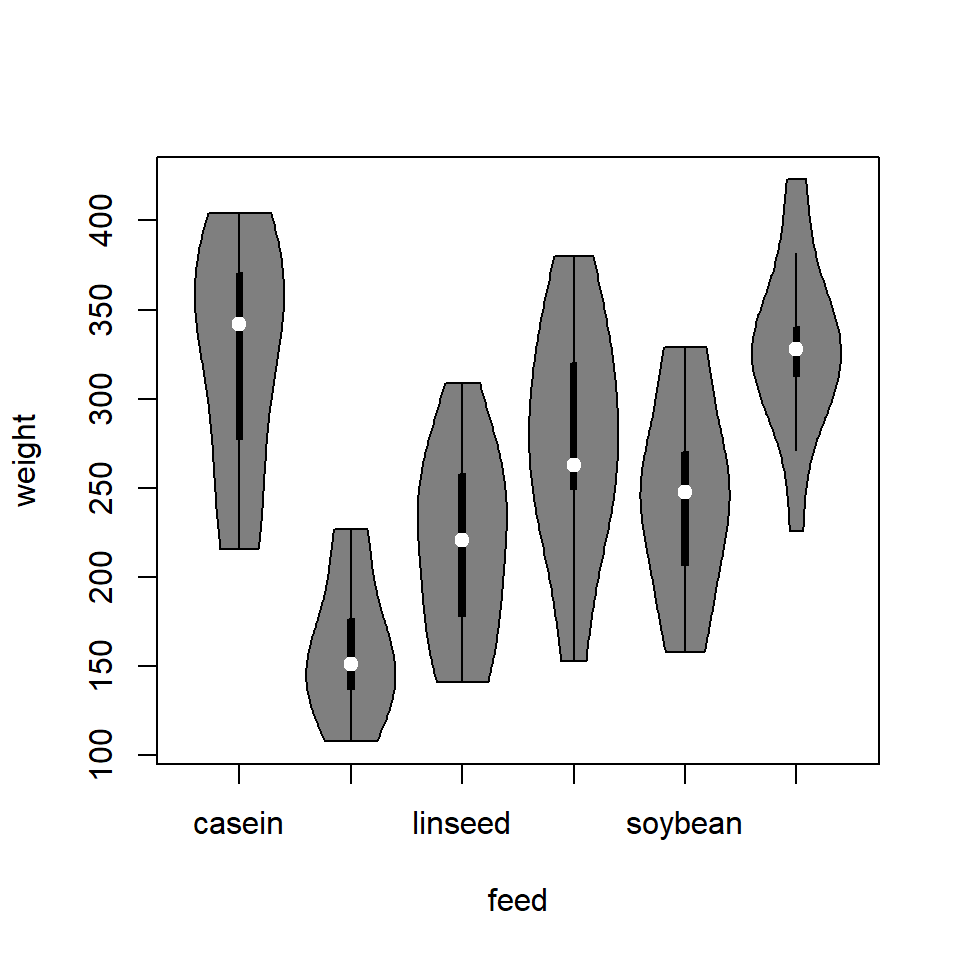Horizontal violin plots

The default violin plots are displayed vertical but it is possible to create a horizontal violin plot setting the `horizontal` argument to `TRUE`.

``````# install.packages("vioplot")
library("vioplot")

vioplot(df\$weight ~ df\$feed,
horizontal = TRUE)``````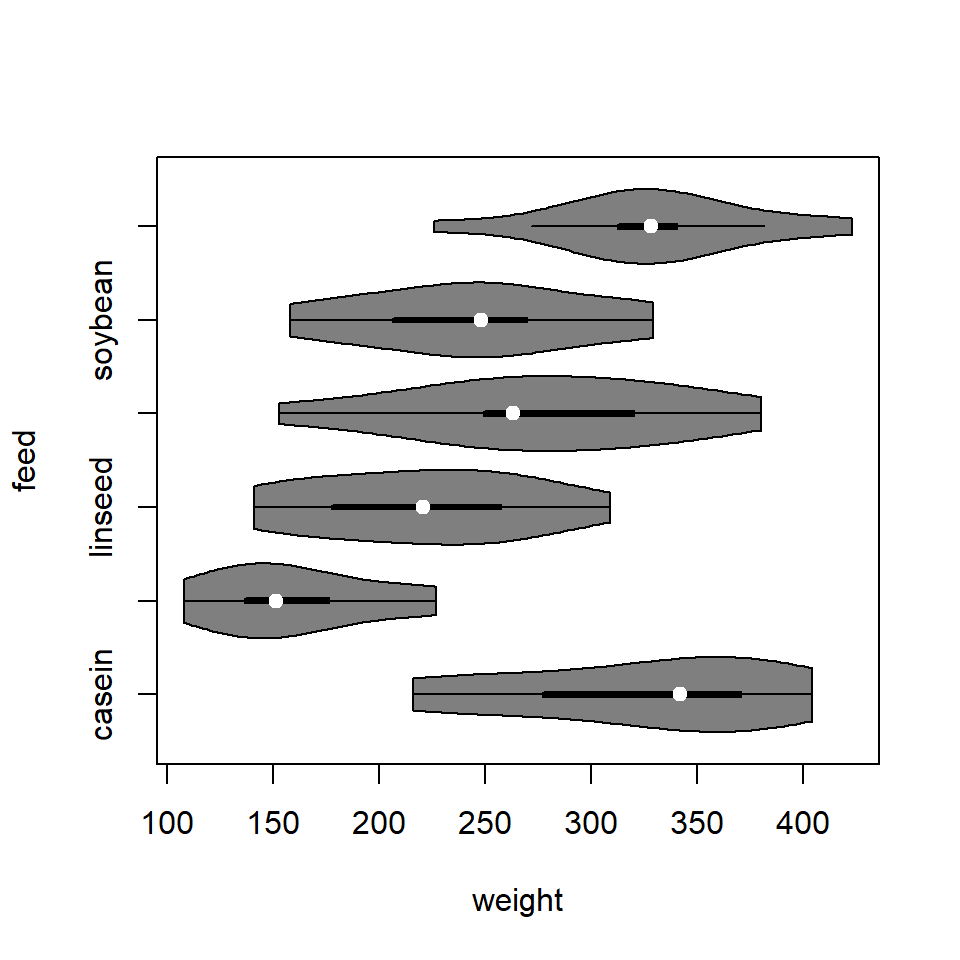Violin sides

You can also plot one side of the violins, setting the argument `side` to `"right"` or `"left"`. Note that both sides are symmetric and represent the kernel density estimate of the underlying data. `vioplot` uses the optimal bandwidth by `sm.density.compare` from `sm` package.

``````# install.packages("vioplot")
library("vioplot")

vioplot(df\$weight ~ df\$feed,
side = "right") # or "left"``````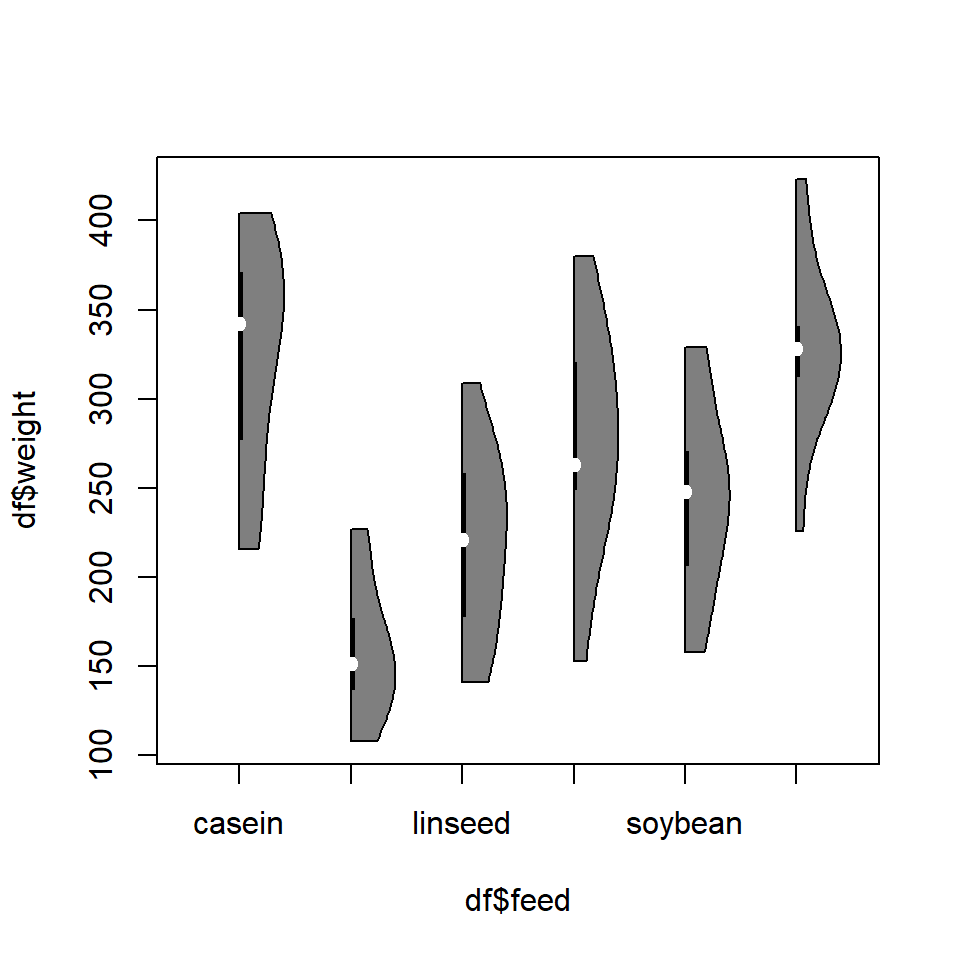## Violin plot colors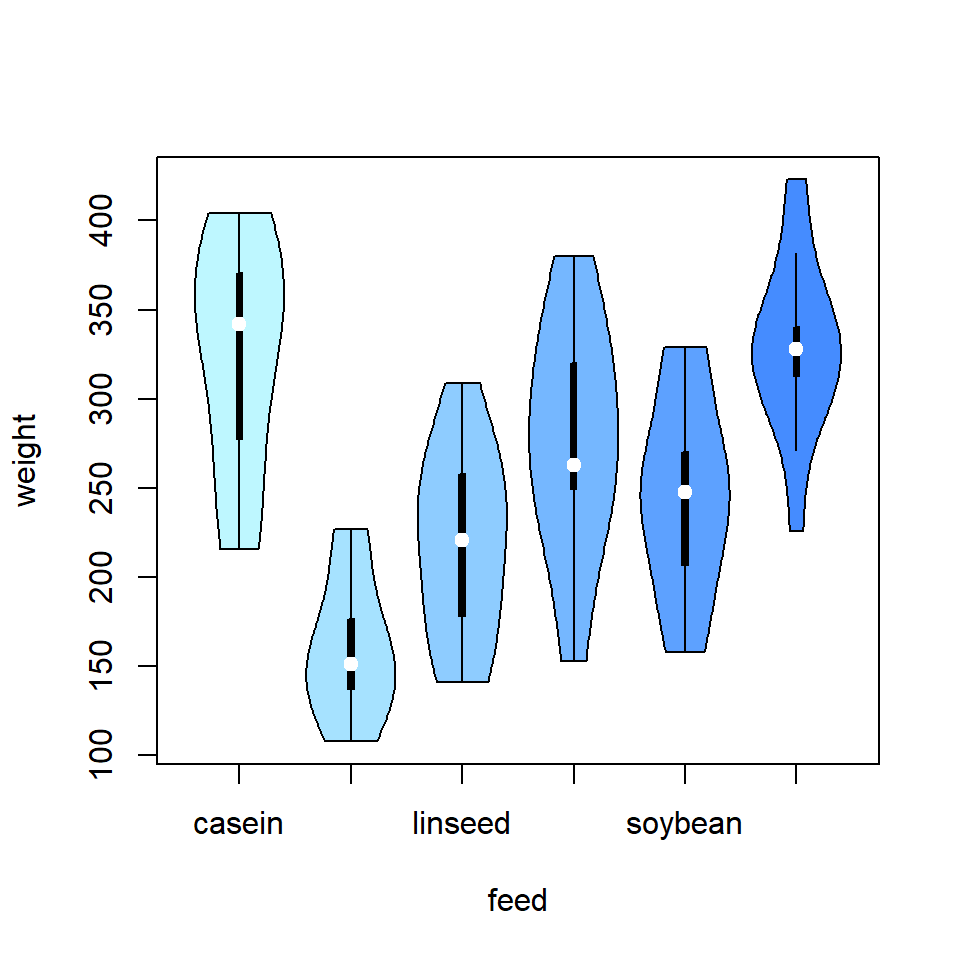Fill color

The colors for each group can be customized with the `col` argument. You can set an unique color to colorize all the violins with the same color or set a vector of colors like in the following example.

``````# install.packages("vioplot")
library("vioplot")

vioplot(weight ~ feed, data = df,
col = c("#bef7ff", "#a6e2ff", "#8eccff",
"#75b7ff", "#5da1ff", "#458cff"))``````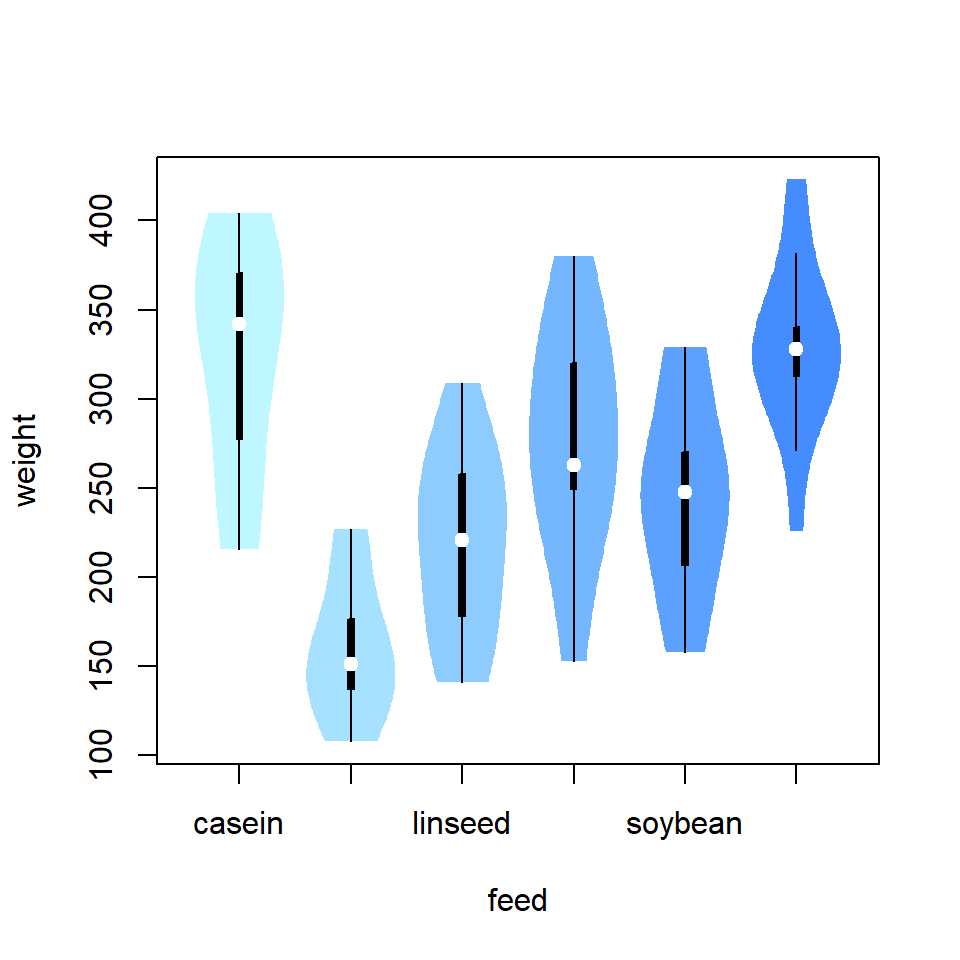Border color

The border for each violin can be customized the same way as the fill color. If you want to remove all the borders set `border = NA`.

``````# install.packages("vioplot")
library("vioplot")

vioplot(weight ~ feed, data = df,
col = c("#bef7ff", "#a6e2ff", "#8eccff",
"#75b7ff", "#5da1ff", "#458cff"),
border = NA)``````